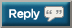1. ## Need Finance HW help bad (7.5k REPS)

any help would be greatly appreciated, reps will be given to anyone who helps

1. TAFKAP Industries has 2.5 million shares of stock outstanding selling at \$21 per share and an issue of \$29 million in 7.3 percent, annual coupon bonds with a maturity of 16 years, selling at 105 percent of par. If TAFKAP's weighted average tax rate is 35 percent and its cost of equity is 14.6 percent.What is TAFKAP's WACC?

8.OMG Inc. has 3.5 million shares of common stock outstanding, 2.9 million shares of preferred stock outstanding, and 5,000 bonds. If the common shares are selling for \$10 per share, the preferred share are selling for \$27 per share, and the bonds are selling for 111 percent of par, what weight, expressed as a percentage, should you use for debt in the computation of OMG's WACC?2.3.4. lol oops i thought it was free5. any help would be greatly appreciated, reps will be given to anyone who helps

1. TAFKAP Industries has 2.5 million shares of stock outstanding selling at \$21 per share and an issue of \$29 million in 7.3 percent, annual coupon bonds with a maturity of 16 years, selling at 105 percent of par. If TAFKAP's weighted average tax rate is 35 percent and its cost of equity is 14.6 percent.What is TAFKAP's WACC?

3.Suppose that B2B, Inc. has a capital structure of 39 percent equity, 17 percent preferred stock, and 44 percent debt. If the before-tax component costs of equity, preferred stock, and debt are 14.4 percent, 12.1 percent, and 9.4 percent, respectively.What is B2B's WACC if the firm faces an average tax rate of 35%?

4. JLP Industries has 7.3 million shares of common stock outstanding with a market price of \$13 per share. The company also has outstanding preferred stock with a market value of \$9.9 million, and 23,000 bonds outstanding, each with a face value of \$1,000 and selling at 94 percent of par value. The cost of equity is 14.1 percent, the cost of preferred is 9.8 percent, and the cost of debt is 7.50 percent. If JLP's tax rate is 30 percent, what is the WACC?

7. OMG Inc. has 8.0 million shares of common stock outstanding, 2.0 million shares of preferred stock outstanding, and 5,000 bonds. If the common shares sell for \$19 per share, the preferred shares sell for \$24 per share, and the bonds sell for 108 percent of par, what weight, expressed as a percentage, should you use for preferred stock in the computation of OMG's WACC?

8.OMG Inc. has 3.5 million shares of common stock outstanding, 2.9 million shares of preferred stock outstanding, and 5,000 bonds. If the common shares are selling for \$10 per share, the preferred share are selling for \$27 per share, and the bonds are selling for 111 percent of par, what weight, expressed as a percentage, should you use for debt in the computation of OMG's WACC?
basic WACC formula: D/V(rD)(1-Tc) + E/V(rE) + P/V(Rp)

1.
E = 52.5 million (2.5 million x \$21)
D = 29 million (market value)
V = 81.5 million (total D+E)
rE = 0.146
rD = .073
Tc (tax) = .35

WACC = (52.5/81.5)*(1-0.35)*0.073 + (29/81.5)*0.146 = 8.26%

3.
e/v = 0.39
p/v = 0.17
d/v = 0.44
rE = 0.144
rP = 0.121
rD = 0.094
Tc = 0.35
WACC = (0.44)*(1-0.35)*0.094 + (0.39)*0.144 + (0.17)*0.121 = 10.36%

V = total firm value (which is value of D+E+P) and then for Debt you always do 1-Tc, for tax shield.6. thanks man will get you on recharge#### Posting Permissions

• You may not post new threads
• You may not post replies
• You may not post attachments
• You may not edit your posts